Algebra 2 6-7 Guided Practice: Inverse Relations and Functions
starstarstarstarstarstarstarstarstarstar
by Matthew Richardson
| 19 Questions1
10
Solve It! What's wrong with the headline? Why? What is a more appropriate headline?

If you decrease an amount x by 20%, you have 0.2x. If you then increase 0.2x by 20%, you get (0.2x)(1.2) = 0.24x, which is less than the original amount x. The headline states that the mayor's salary was restored, but the mayor's salary will be 76% less than the original salary. A more appropriate headline would be Some of Mayor's Salary Restored.
If you decrease an amount x by 20%, you have 0.8x. If you then increase 0.8x by 20%, you get (0.8x)(1.2) = 0.96x, which is less than the original amount x. The headline states that the mayor's salary was restored, but the mayor's salary will be 4% less than the original salary. A more appropriate headline would be Most of Mayor's Salary Restored.2
2
10
Take Note: What is an inverse function ?
3
10
Problem 1 Got It? What are the graphs of t and its inverse? Represent both relations as mapping diagrams on the canvas.

4
10
Problem 1 Got It? Reasoning: Refer to the mapping diagrams you created in the previous item.

Is t a function? Is the inverse of t a function? Explain.
t is a function; the inverse of t is not a function because there are two y-values for one x-value.
t is not a function; the inverse of t is also not a function; in both t and its inverse, there are two y-values for at least one x-value.
t is a function; the inverse of t is also a function because there is only one y-value for each x-value.
t is a function; the inverse of t is also a function: in both t and its inverse, there is only one y-value for each x-value.5
5
10
Take Note: In general, what is the process for finding the inverse of a given equation.6
6
10
Problem 2 Got It?
A
B
C
D
7
10
Problem 3 Got It? Graph the function, its inverse, and the line y = x on the same plane.
Note that the function and its inverse should be reflections of each other across the line y = x.
Zoom and pan your graph to establish an appropriate viewing window.8
8
10
Take Note: How are the domain and range of a function and the domain and range of the function's inverse related?9
9
10
Problem 4 Got It?
A
B
C
D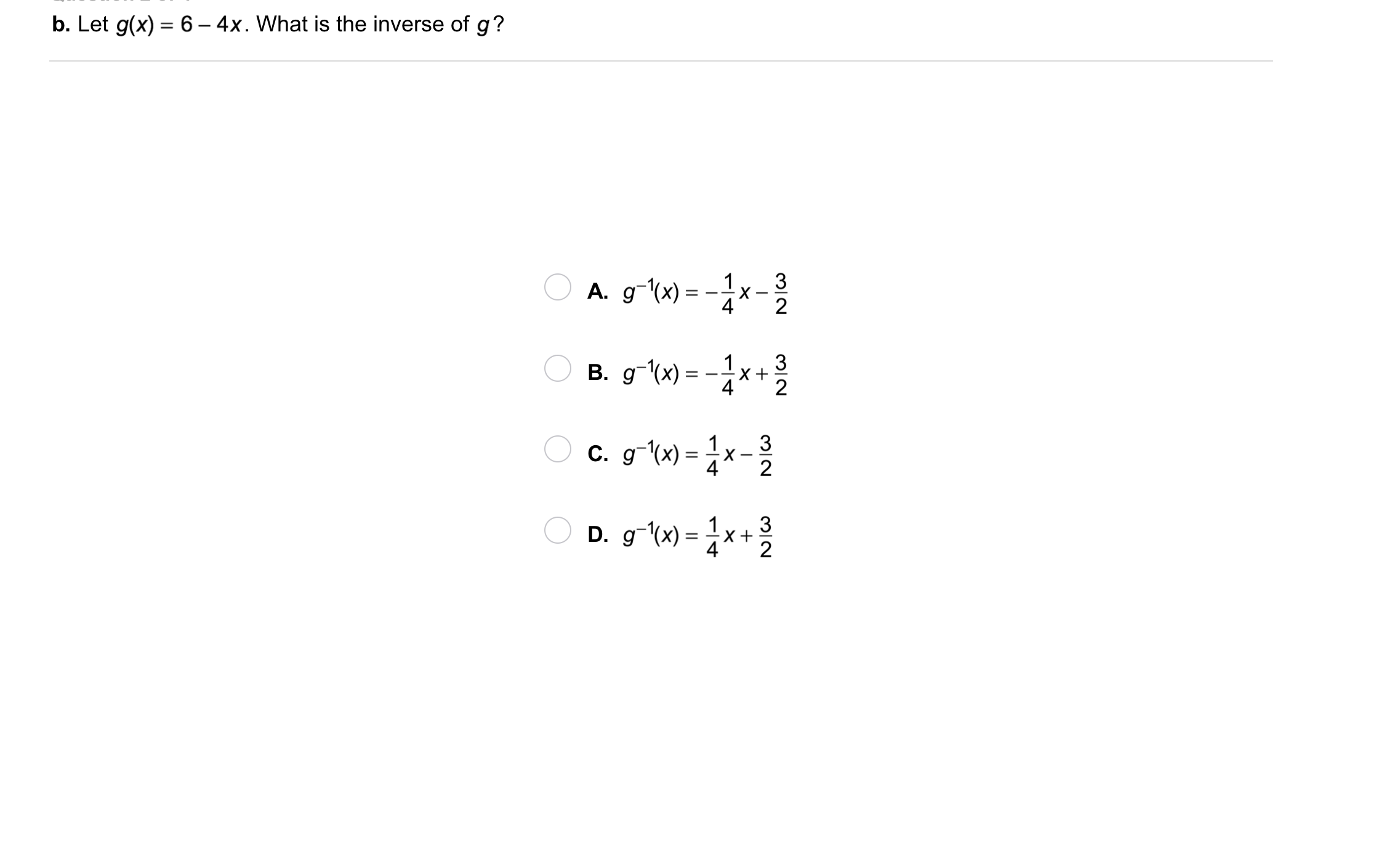10
10
10
Problem 4 Got It?
A
B
C
D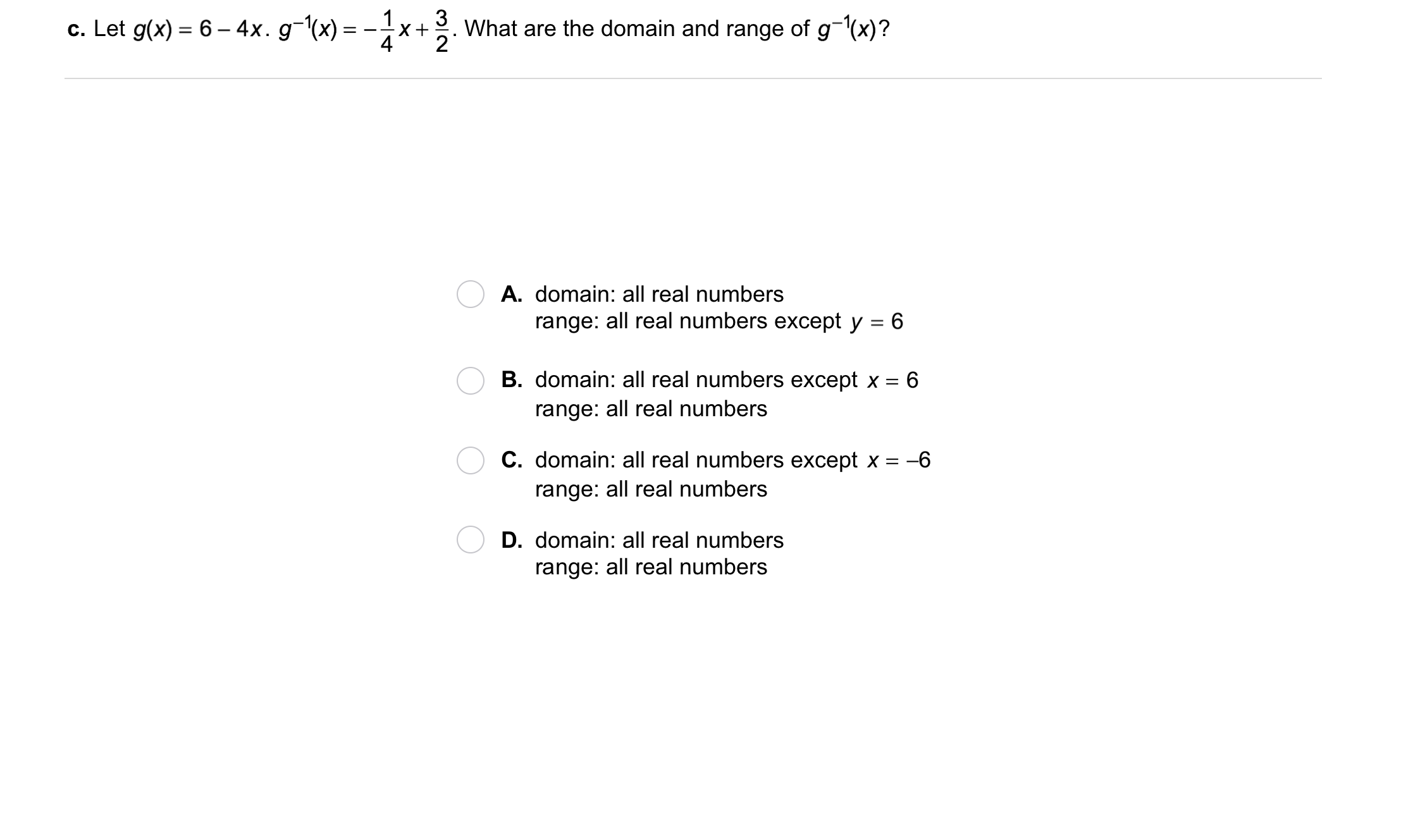11
11
10
Problem 4 Got It?
A
B
C
D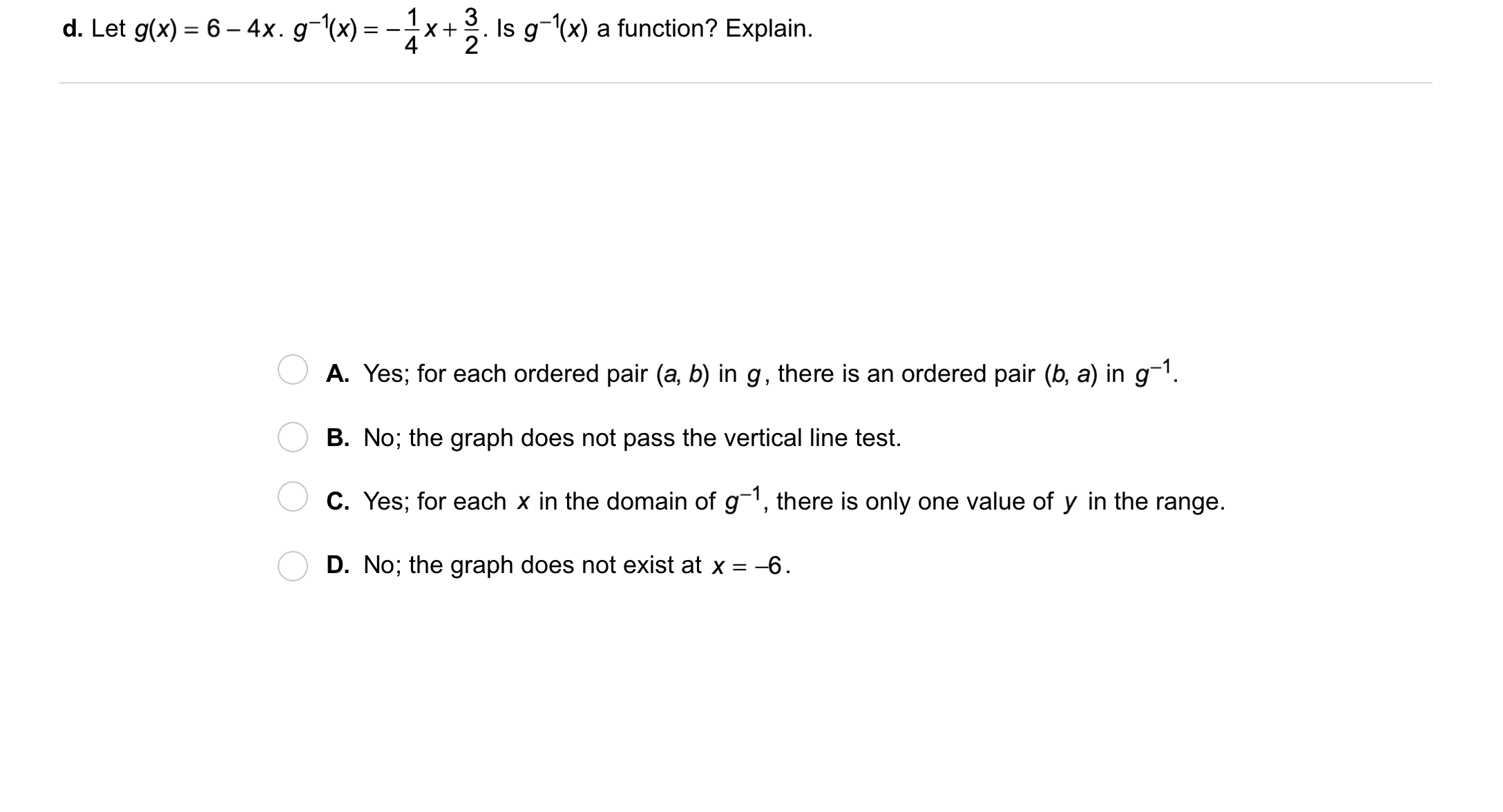12
12
10
Problem 4 Got It?
A
B
C
D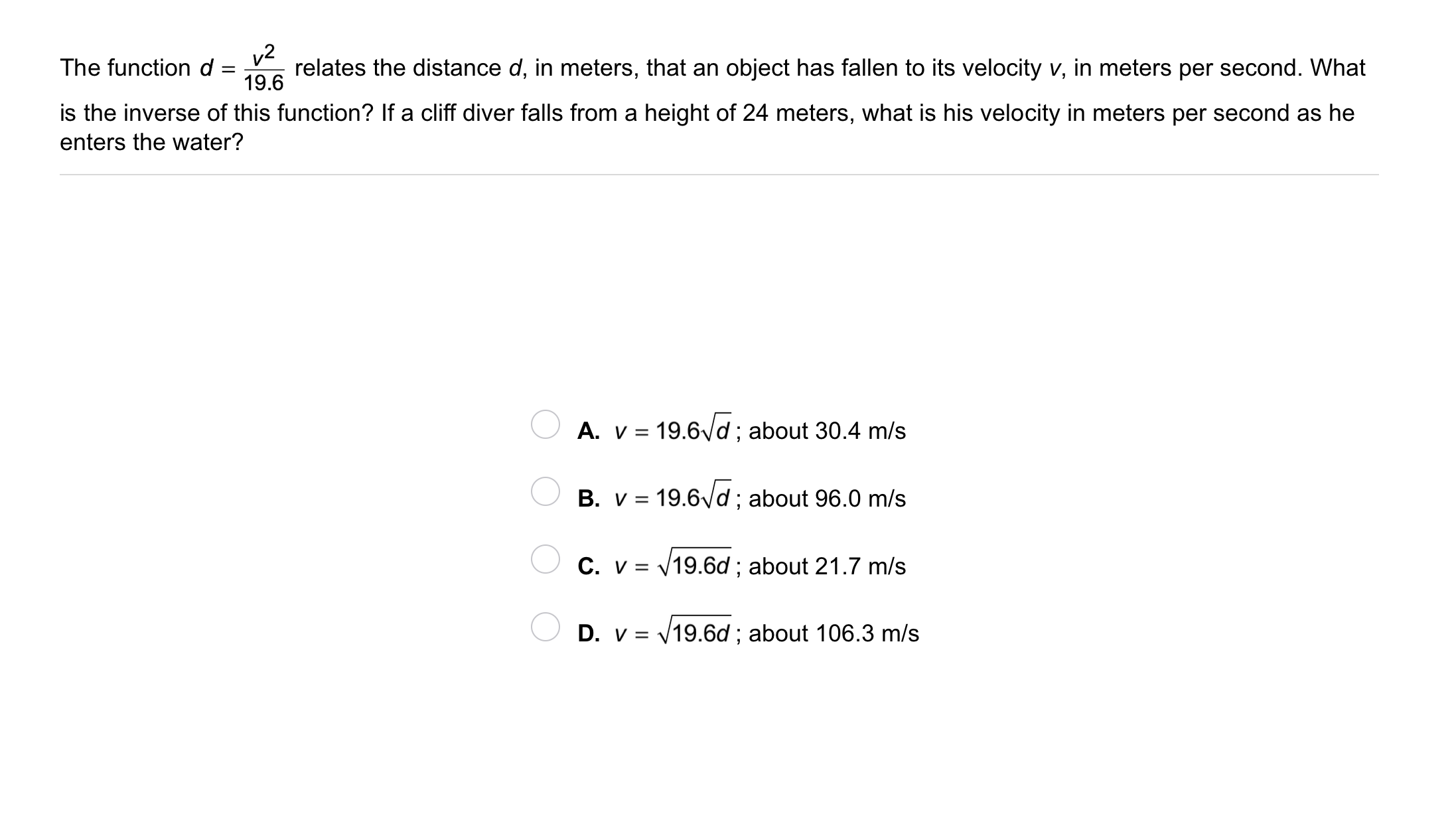13
13
10
Problem 5 Got It? The function d, below, relates the distance d, in meters, that an object has fallen to its velocity, v, in meters per second.
What is the inverse of this function?

If a cliff diver falls from a height of 24 meters, what is his velocity in meters per second as he enters the water?
A
B
C
D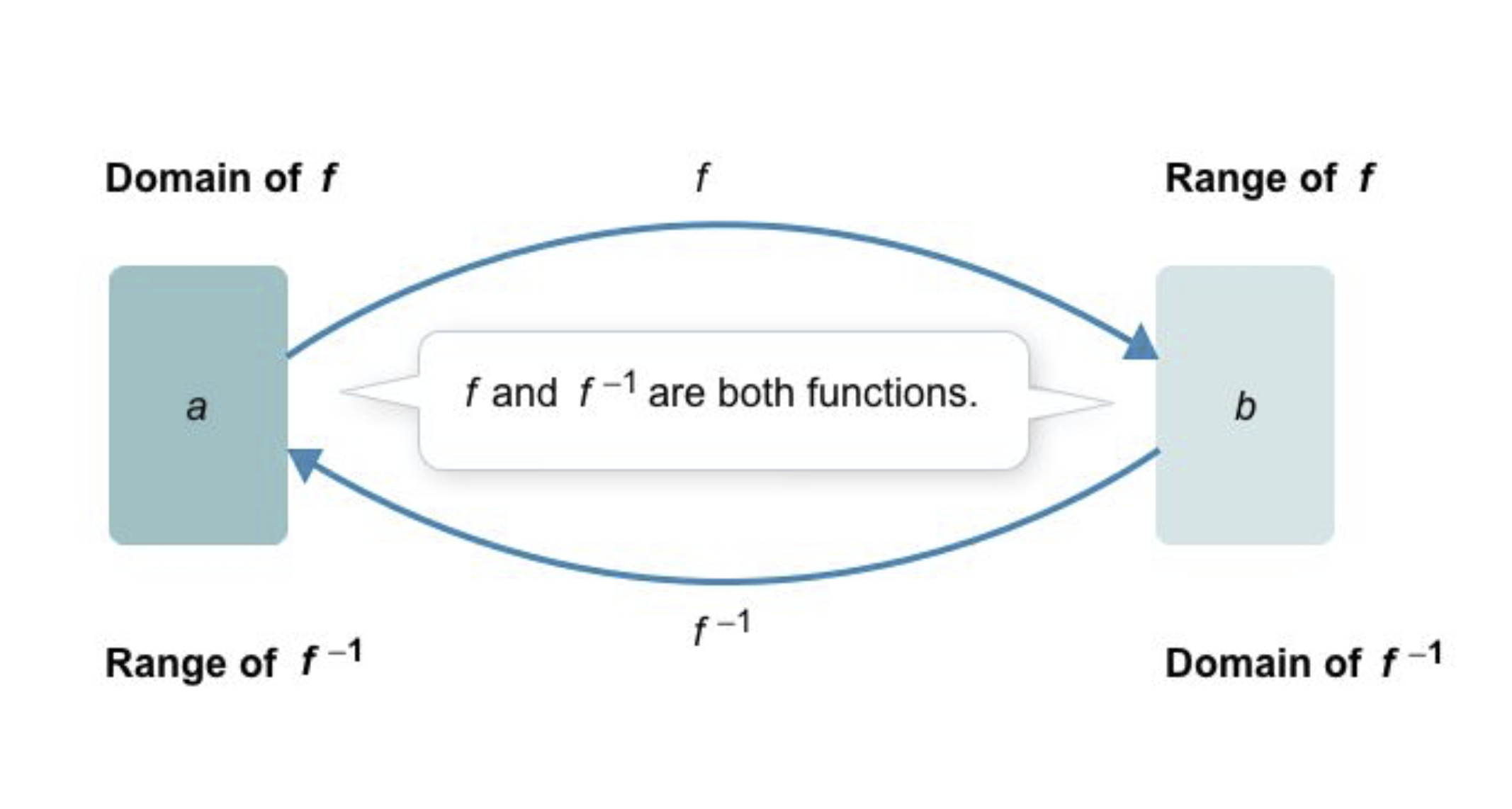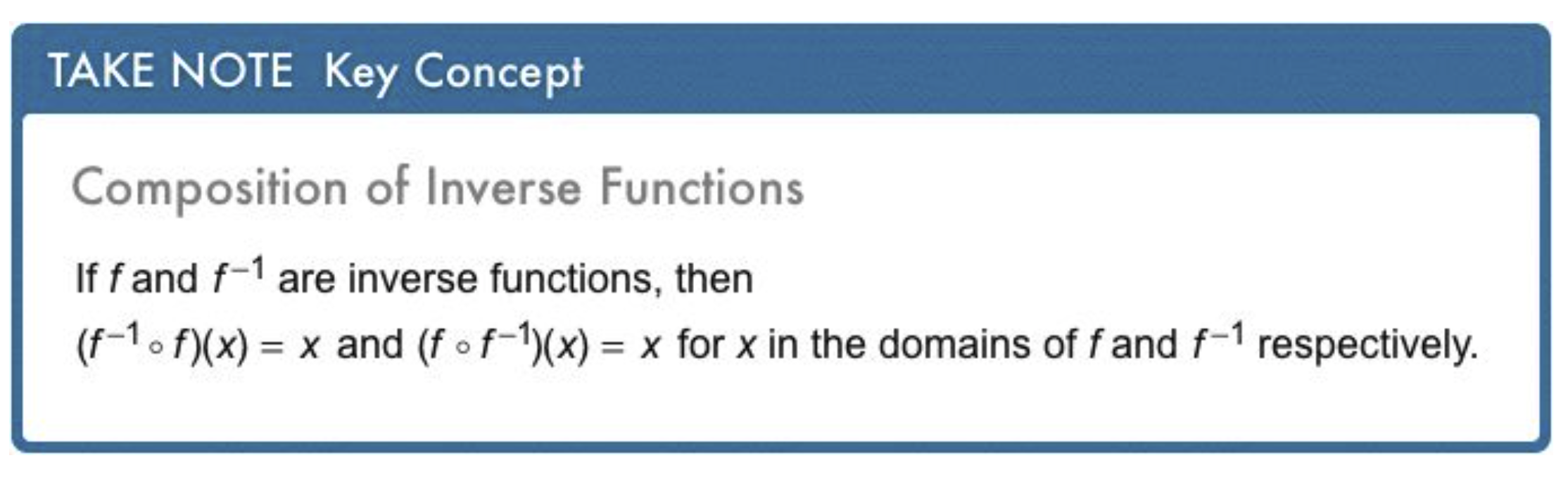14
15
14
10
Take Note: What is a one-to-one function ?
15
10
Take Note: What happens when you compose a function and its inverse?

For example, consider:

16
10
Problem 6 Got It? Given g(x) below, find g-1(x).17
17
10
Problem 6 Got It?
A
B
C
D18
18
10
Problem 6 Got It?
A
B
C
D19
19
10
Take Note: Summarize the content of this lesson.